# Statistics and Probability (Part - 2) Notes | Study Quantitative Reasoning for GMAT - GMAT

## GMAT: Statistics and Probability (Part - 2) Notes | Study Quantitative Reasoning for GMAT - GMAT

The document Statistics and Probability (Part - 2) Notes | Study Quantitative Reasoning for GMAT - GMAT is a part of the GMAT Course Quantitative Reasoning for GMAT.
All you need of GMAT at this link: GMAT

FREQUENCY POLYGON
A frequency polygon is a graph of frequency distribution. It is a line graph of class frequency which is plotted against class mark. A frequency polygon can be obtained by two methods:
(1) By using Histogram: A frequency polygon can be obtained by joining mid points of the top of the rectangles of a histogram. For this we obtain the mid points of the upper horizontal sides of each rectangle and then join these mid points by dotted lines to get frequency polygon. End of a frequency polygon preferably extended to the mid points of imagined class intervals adjacent to first and last class intervals.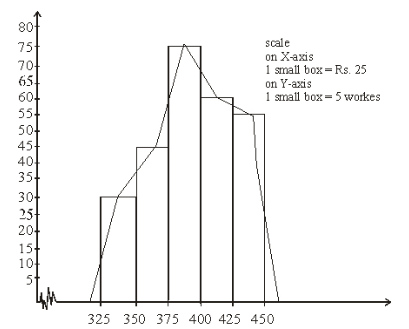(2) Frequency polygon without using Histogram: Following procedure is used to make a frequency polygon without using histogram.
(i)  Calculate the class marks, x1, x2, ...., xn of each of the given class intervals.
(ii) Mark class marks x1, x2, .... xn, along X-axis and frequencies f1, f2, .... fn along Y-axis.
iii) Plot the points (x1, f1), (x2, f2), ,....., (xn, fn).
(iv) Obtain the mid-points of two class intervals of zero frequencies at the beginning of the first interval and at the end of the last interval.
(v) Join the points (x1, f1), (x2, f2), ..., (xn, fn) by the line segments and complete the frequency polygon by joining the mid points of the first and last intervals to the mid points of the imagined classes adjacent to them.

CUMULATIVE FREQUENCY CURVE OR OGIVE
The graphical representation of a cumulative frequency distribution is known as cumulative frequency curve or an ogive.
An ogive can be constructed by following two methods:
(1) Less than method: A less than ogive can be constructed by following steps:
(i) First of all we make class intervals in exclusive form if it is given in inclusive form.
(ii) Then we construct a less than type cumulative frequency distribution by adding the frequency of each class to the sum of frequencies of its prior classes.
(iii) Now we mark upper class limits along X-axis and cumulative frequencies along Y-axis.(iv) We plot the points (upper class limit, corresponding cumulative frequency) and join them by a free hand curve.
(v) The lower limit of the first class interval becomes the upper limit of the imagined class with frequency 0. We join the imagined point (lower limit of first class, 0) with the first point of the curve and so on.
In this way we get the required curve called an Ogive by less than type method.

More than Type:
We apply the following steps to construct a more than type ogive:
Step (1)    :   First of all we make class intervals in exclusive form if it is given in inclusive form.
Step (2)    :   Then we construct a more than type cumulative frequency distribution.
Step (3)    :   Now we mark lower lass limits along x-axis and cumulative frequencies along y-axis.
Step (4)    :  We plot the points (lower class limit, corresponding cumulative frequency) and join them by a free hand curve.
Step (5)    :   The upper limit of the last class interval becomes the lower limit of the imagined class interval with frequency 0. We join the imagine point (upper limit of last class, 0) with the last point of the curve to end the ogive.
In this way we get the required curve called an ogive by more than type method.

MEASURES OF CENTRAL TENDENCY
An average of a distribution is a single expression which represents a group of variables in a simple and concise manner. It is the representative of entire distribution. Averages are generally in the central parts of the distribution and therefore they are called Measures of Central Tendency.
An ideal measures of central tendency should have following properties:
(i) It should be defined rigidly.
(ii) It should be based on all observations.
(iii) It should be easy to calculate and readily comprehensible.
(iv) It should be affected as less as possible by fluctuations of sampling.
(v) Extreme values should not affect very much to measure of central tendency.
Following three types of measures of central tendency are used for analysing data:
(i)   Arithmetic mean
(ii)  Median
(iii) Mode
Arithmetic mean for ungrouped data (A. M.)
The arithmetic mean is the most commonly used measure of central tendency. It is obtained by dividing number of observations to the sum of observations. The A. M.  of n observations, x1, x2, x3, ......,, xn is given by
A.M =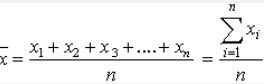Properties of Arithmetic Mean
(1) If x is the mean of n observations, x1, x2, ....., xn, then the mean of observations x1 + a, x2 + a, ...., xn + a is , i.e. if each observation is increased by a, then the mean is also increased by a.
(2) If  is the mean of n observations, x1, x2, ..... xn, then the mean of observation, x1 – a, x2 – a, ..., xn – a is  i.e. if each observation is decreased by a, then the mean is also decreased by a.
(3) If  is the mean of x1, x2, .... xn then mean of ax1, ax2, .... axn is , where a is any number different from zero i.e. if each observation is multiplied by a non-zero number a, then the mean is also multiplied by a.
(4) If  is the mean of n observations x1, x2, ...., xn then the mean of x1/a, x2/a, ..... xn/a is xÌ„/a where a ≠ 0,  i.e. if each observation is divided by a non-zero number, then the mean is also divided by it.

Arithmetic mean of Grouped Data:
Let x1, x2, x3, ..... xn be n observations whose frequencies are f1, f2, f3, .., fn respectively, then the arithmetic mean of this distribution is given by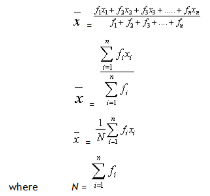Combined Mean
Let  and  be the means of two groups of observations with number of observations n1 and n2 respectively, then the combined mean of two groups is given by,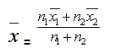Merits of Arithmetic Mean
(i) A. M. is rigidly defined.
(ii) It is very simple. One can easily understand and calculate it.
(iii) It is uniquely defined.
(iv) It is based upon all the observations.
(v)  A. M. is least affected by sampling fluctuations.
(vi) We can mathematically analysis mean.
(vii) A. M. relatively reliable.

Demerits of Arithmetic Mean
(i) A. M. cannot be used for qualitative characteristics like richness, beauty, poverty etc.
(ii)  A. M. of a given data can not be determined by inspection. It can be also represented graphically also.
(iii) If any observation is missing then A.M. cannot be calculated.
(iv) A. M. is very much affected by extreme values. In case of extreme items, A. M. gives a distorted picture of the distribution and no longer remains representative of the distribution.
(v)  If the extreme class is open, e.g. below 10 or above 100 then A. M. cannot be calculated.
(vi) If the given data from which the mean has to be calculated, is not given then A. M. may lead to wrong conclusions.
(vii) A. M. cannot be used in the study of ratios, rates etc.

Uses of Arithmetic Mean
(i) A. M. is extensively used in practical statistics.
(ii)  Estimates can be obtained using A. M.
(iii) A. M. is used for different purposes by different persons like it is used for calculating average marks of the students. It is also used by businessmen to find out profit per unit article, output per machine, average monthly income and expenditure etc.
=  16 – 6
=  10
Hence, f1 = 6 and     f2 = 10

MEDIAN
Median is defined as the value of that item of the arrayed data which divides the whole data into two equal parts. Hence we have following definition of median:

The middle item of the arrayed data is called its median.

Calculation of median of raw data:
(i)   If the number of observations ‘n’ is odd, then the median will be the value of  observation.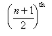(ii) If n is even, then we have two middle terms i.e. (n/2)th observation and  (n/2 + 1)th observation.
Median of the given data will be mean of these two middle observations.

The document Statistics and Probability (Part - 2) Notes | Study Quantitative Reasoning for GMAT - GMAT is a part of the GMAT Course Quantitative Reasoning for GMAT.
All you need of GMAT at this link: GMATUse Code STAYHOME200 and get INR 200 additional OFF

## Quantitative Reasoning for GMAT

75 videos|87 docs|156 tests

Track your progress, build streaks, highlight & save important lessons and more!

,

,

,

,

,

,

,

,

,

,

,

,

,

,

,

,

,

,

,

,

,

;